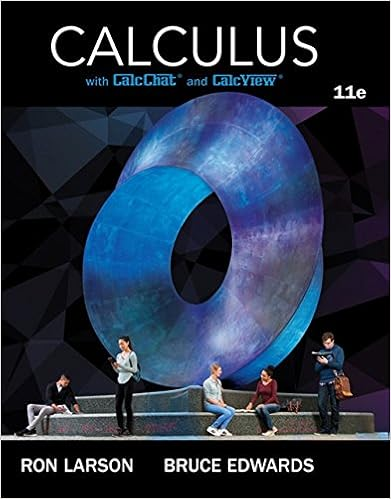# 34 correct correct answers 075 17 4 pts the series n

• Homework Help
• 6

This preview shows page 5 - 6 out of 6 pages.

##### We have textbook solutions for you!
The document you are viewing contains questions related to this textbook.The document you are viewing contains questions related to this textbook.
Chapter 9 / Exercise 71
Calculus
Edwards/LarsonExpert Verified
3/4 (correct) Correct Answers: 0.75 17. (4 pts) The series n = 1 n k r n converges when 0 < r < 1 and diverges when r > 1 . This is true regardless of the value of the constant k . When r = 1 the series is a p-series. It converges if k < - 1and diverges otherwise. Each of the series below can be compared to a series of the form n = 1 n k r n . For each series deter- mine the best value of r and decide whether the series converges. A. n = 1 ( 5 + n ( 6 ) n ) - 3 r = converges or diverges (c or d)? B. n = 1 n π 3 2 n 4 n + n 9 r = converges or diverges (c or d)? C. n = 1 n . 5 + 4 n 4 + 6 r = converges or diverges (c or d)? D. n = 1 4 n 2 + 3 n + 5 - 5 n 9 n + 5 + 4 n + 6 n 5 5
##### We have textbook solutions for you!
The document you are viewing contains questions related to this textbook.The document you are viewing contains questions related to this textbook.
Chapter 9 / Exercise 71
Calculus
Edwards/LarsonExpert Verified
r = converges or diverges (c or d)?
6
•••